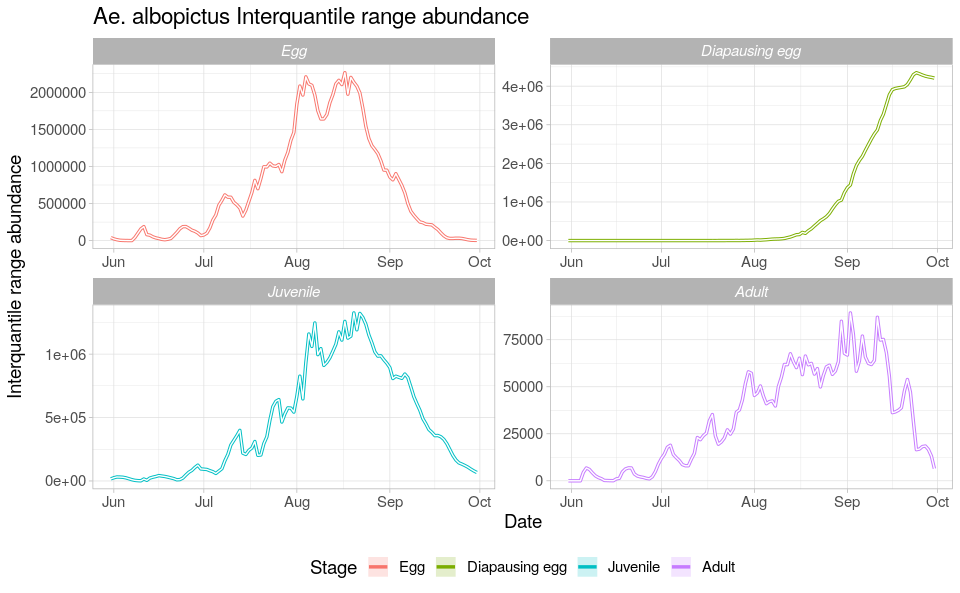# Describing the main features of dynamAedes

#### 2022-01-16

This tutorial explains step-by-step the main features of dynamAedes package, a unified modelling framework for invasive Aedes mosquitoes. Users can apply the stochastic, time-discrete and spatially-explicit population dynamical model initially developed here https://doi.org/10.1016/j.ecoinf.2020.101180 for Aedes aegypti and then expanded for other three species: Ae. albopictus, Ae. japonicus and Ae. koreicus here: http://dx.doi.org/10.1101/2021.12.21.473628 .

The model is driven by temperature, photoperiod and intra-specific larval competition and can be applied to three different spatial scales: punctual, local and regional. These spatial scales consider different degrees of spatial complexity and data availability, by accounting for both active and passive dispersal of the modelled mosquito species as well as for the heterogeneity of input temperature data.

We will describe model applications for Ae. albopictus and for all spatial scales by using a simulated temperature dataset.

#Load packages
require(spatstat)
require(sp)
require(gstat)
require(parallel)
require(eesim)
require(tidyverse)
require(geosphere)
require(ggplot2)
require(rgeos)
require(dynamAedes)

# Punctual scale model

At punctual scale, the model only requires a weather station temperature time series provided as a numerical matrix (in degree Celsius). For the purpose of this tutorial, we simulate a 1-year long temperature time series.

## Simulate temperature data with seasonal trend

We first simulate a 1-year temperature time series with seasonal trend. For the time series we consider a mean value of 16°C and standard deviation of 2°C.

ndays = 365*1 #length of the time series in days
set.seed(123)
sim_temp <- create_sims(n_reps = 1,
n = ndays,
central = 16,
sd = 2,
exposure_type = "continuous",
exposure_trend = "cos1", exposure_amp = -1.0,
average_outcome = 12,
outcome_trend = "cos1",
outcome_amp = 0.8,
rr = 1.0055)

A visualisation of the distribution of temperature values and temporal trend.

hist(sim_temp[]$x, xlab="Temperature (°C)", main="Histogram of simulated temperatures")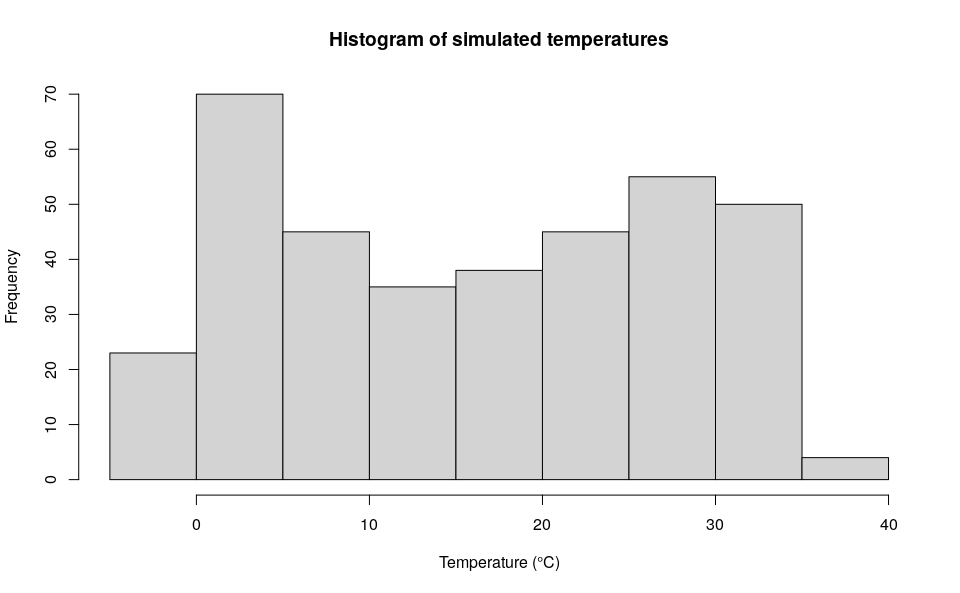plot(sim_temp[]$date,
sim_temp[]$x, main="Simulated temperatures seasonal trend", xlab="Date", ylab="Temperature (°C)" )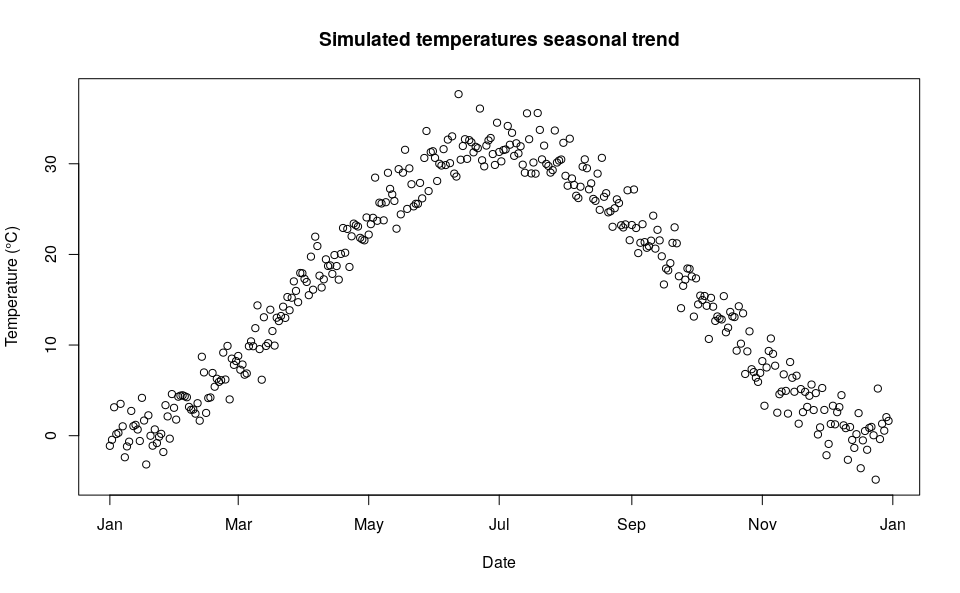## Format the simulated input datasets and run the model ### Model settings Float numbers in the temperature matrix would slow the computational speed, they must be multiplied by 1000 and then transformed in integer numbers. We also transpose the matrix from long to wide format, since we conceptualised the model structure considering the rows as the spatial component (e.g. observations; here = 1) and the columns as the temporal one (e.g. variables). df_temp <- data.frame("Date" = sim_temp[]$date, "temp" = sim_temp[]$x) w <- t(as.integer(df_temp$temp*1000))

We are now left with a few model parameters which need to be defined by the user.

## Define the day of introduction (August 1st is day 1)
str = 213
## Define the end-day of life cycle (September 1st is the last day)
endr = 213+31
## Define the number of eggs to be introduced
ie = 100
## Define the number of model iterations
it = 1 # The higher the number of simulations the better
## Define the number of liters for the larval density-dependent mortality
habitat_liters=1
#Define latitude and longitude for the diapause process
myLat=42
myLon=7
## Define the number of parallel processes (for sequential itarations set nc=1)
cl = 1
## Set output name for the *.RDS output will be saved
#outname= paste0("dynamAedes_albo_ws_dayintro_",str,"_end",endr,"_niters",it,"_neggs",ie)

### Run the model

Running the model with the settings specified in this example takes about 2 minutes.

simout <- dynamAedes(species="albopictus",
scale="ws",
ihwv=habitat_liters,
temps.matrix=w,
startd=str,
endd=endr,
n.clusters=cl,
iter=it,
intro.eggs=ie,
compressed.output=TRUE,
lat=myLat,
long=myLon,
verbose=FALSE,
seeding=TRUE)

## Analyse the results

We first explore the model output structure: the simout object is a nested list.

The first level corresponds to the number of model iterations

print(it)
#  1
print(length(simout))
#  1

The second level corresponds to the simulated days. So if we inspect the first iteration, we observe that the model has computed 62 days, as we had specified above in the object endr.

length(simout[])
#  32

The third level corresponds to the amount of individuals for each stage (rows). So if we inspect the 1st and the 62th day within the first iteration, we obtain a matrix having

dim(simout[][])
#  4 1
simout[][]
#              [,1]
# egg           100
# juvenile       34
# diapause_egg    0
simout[][]
#              [,1]
# egg           783
# juvenile     1726
# diapause_egg    0

We can now use the auxiliary functions of the package to analyse the results.

### Derive probability of a successfull introduction at the end of the simulated period

First, we can retrieve the “probability of successful introduction”, computed as the proportion of model iterations that resulted in a viable mosquito population at a given date.

psi(input_sim = simout, eval_date = 31)
#   Days_after_intro p_success      stage
# 1           Day 31         1 Population

### Derive abundance 95% CI for each life stage and in each day

We now compute the interquantile range abundance for all the stages of the simulated population using the function adci.

dd <- max(sapply(simout, function(x) length(x)))#retrieve the maximum number of simulated days
egg <- as.data.frame(adci(simout, eval_date=1:dd, breaks=c(0.25,0.50,0.75), st=1))
juv <- as.data.frame(adci(simout, eval_date=1:dd, breaks=c(0.25,0.50,0.75), st=2))
eggd <- as.data.frame(adci(simout, eval_date=1:dd, breaks=c(0.25,0.50,0.75), st=4))

egg$myStage="Egg" egg$Date=seq.Date(sim_temp[]$date[str], sim_temp[]$date[endr], by="day")
juv$myStage="Juvenile" juv$Date=seq.Date(sim_temp[]$date[str], sim_temp[]$date[endr], by="day")
ad$myStage="Adult" ad$Date=seq.Date(sim_temp[]$date[str], sim_temp[]$date[endr], by="day")
eggd$myStage="Diapausing egg" eggd$Date=seq.Date(sim_temp[]$date[str], sim_temp[]$date[endr], by="day")

as_tibble()

outdf %>%
mutate(myStage=factor(myStage, levels= c("Egg", "Diapausing egg", "Juvenile", "Adult"))) %>%
ggplot( aes(y=(50%),x=Date, group=factor(myStage),col=factor(myStage))) +
ggtitle("Ae. albopictus Interquantile range abundance")+
geom_line(size=1.2)+
geom_ribbon(aes(ymin=25%,ymax=(75%),fill=factor(myStage)),
col="white",
alpha=0.2,
outline.type="full")+
labs(x="Date", y="Interquantile range abundance", col="Stage", fill="Stage")+
facet_wrap(~myStage, scales = "free")+
theme_light()+
theme(legend.pos="bottom",  text = element_text(size=14) , strip.text = element_text(face = "italic"))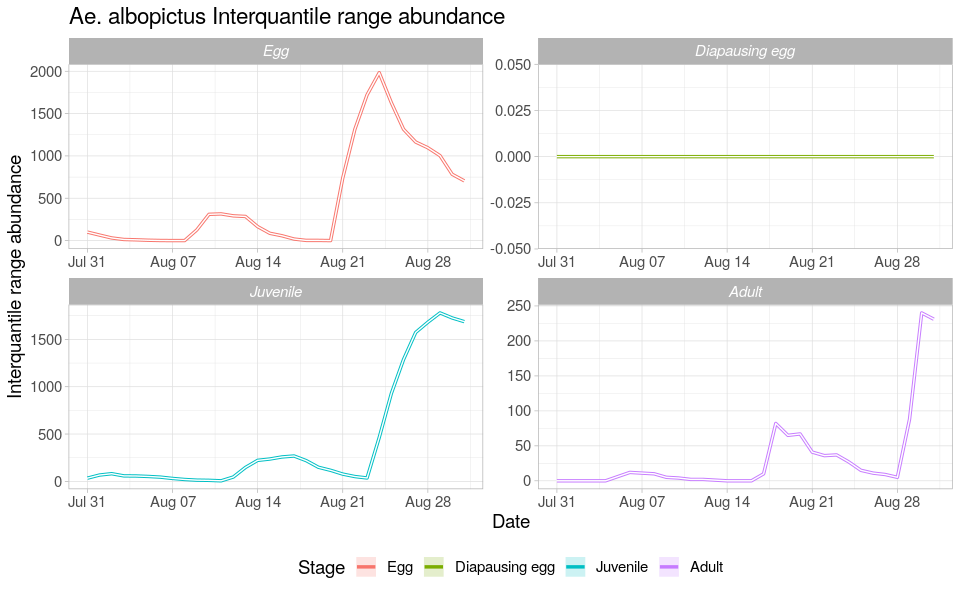# Local scale model The “local scale” means that the model accounts for both active and passive dispersal of the mosquitoes. With this setting, the model requires three input datasets: a numerical temperature matrix (in degree Celsius) defined in space and time (space in the rows, time in the columns), a two-column numerical matrix reporting the coordinates (in meters) of each space-unit (cell) and a numerical distance matrix which reports the distance in meters between the cells connected through a road network. For the purpose of this tutorial, we will use the following simulated datasets:

1. A 5 km lattice grid with 250 m cell size;
2. A 1-year long spatially and temporally correlated temperature time series;
3. A matrix of distances between cells connected through a simulated road network;

## Prepare input data

### Create lattice arena

First, we define the physical space into which the introduction of our mosquitoes will happen. We define a squared lattice arena having 5 km side and 250 m resolution (20 colums and 20 rows, 400 total cells).

gridDim <- 20 # 5000m/250 m = 20 columns and rows
xy <- expand.grid(x=1:gridDim, y=1:gridDim)

We then add a spatial pattern into the lattice area. This spatial pattern will be used later to add spatial correllation (SAC) to the temperature time series. The spatial autocorrelated pattern will be obtained using a semivariogram model with defined sill (value that the semivarion attains at the range) and range (distance of 0 spatial correlation) and then predicting the semivariogram model over the lattice grid using unconditional Gaussian simulation.

varioMod <- vgm(psill=0.005, range=100, model='Exp') # psill = partial sill = (sill-nugget)
# Set up an additional variable from simple kriging
zDummy <- gstat(formula=z~1,
locations = ~x+y,
dummy=TRUE,
beta=1,
model=varioMod,
nmax=1)
# Generate a randomly autocorrelated predictor data field
set.seed(123)
xyz <- predict(zDummy, newdata=xy, nsim=1)
# [using unconditional Gaussian simulation]

We generate a spatially autocorrelated raster adding the SA variable (xyz$sim1) to the RasterLayer object. The autocorrelated surface could for example represent the distribution of vegetation cover in a urban landscape. utm32N <- "+proj=utm +zone=32 +ellps=WGS84 +datum=WGS84 +units=m +no_defs" r <- raster(nrow=gridDim, ncol=gridDim, crs=utm32N, ext=extent(0,5000, 0,5000)) values(r)=xyz$sim1
plot(r)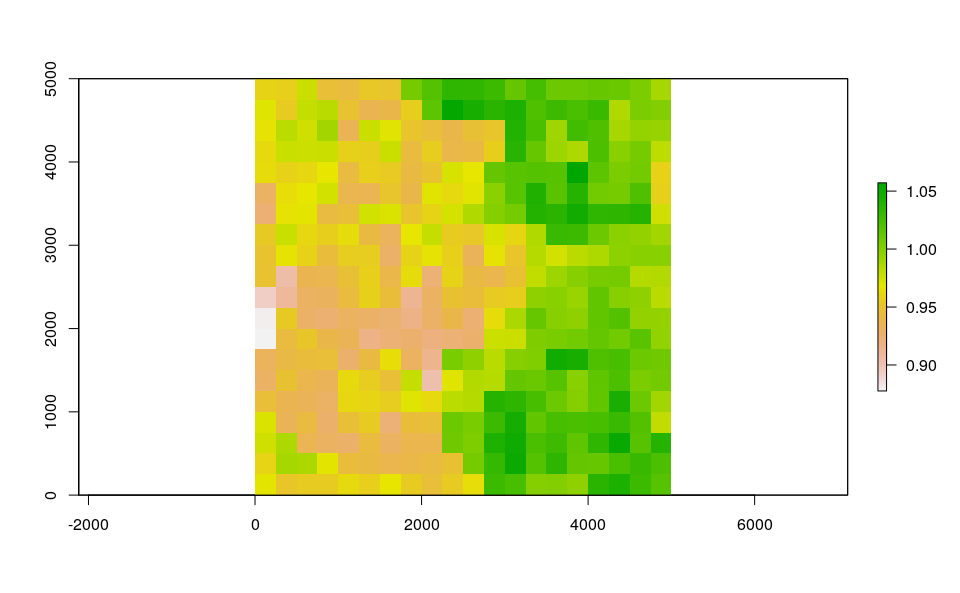df <- data.frame("id"=1:nrow(xyz), coordinates(r))
bbox <- as(extent(r), "SpatialPolygons")

# Store Parameters for autocorrelation
autocorr_factor <- values(r)

### Simulate temperature data with seasonal trend

We take advantage of the temperature dataset simulated for the punctual scale modelling exercise. We can then “expand onto space” the temperature time series by multiplying it with the autocorrelated surface simulated above.

mat <- lapply(1:ncell(r), function(x) {
d_t <- sim_temp[]$x*autocorr_factor[[x]] return(d_t) }) mat <- do.call(rbind,mat) A comparison between the distribution of the initial temperature time series and autocorrelated temperature surface par(mfrow=c(2,1)) hist(mat, xlab="Temperature (°C)", main="Histogram of simulated spatial autocorreled temperature") hist(sim_temp[]$x, xlab="Temperature (°C)", main="Histogram of simulated temperatures", col="red")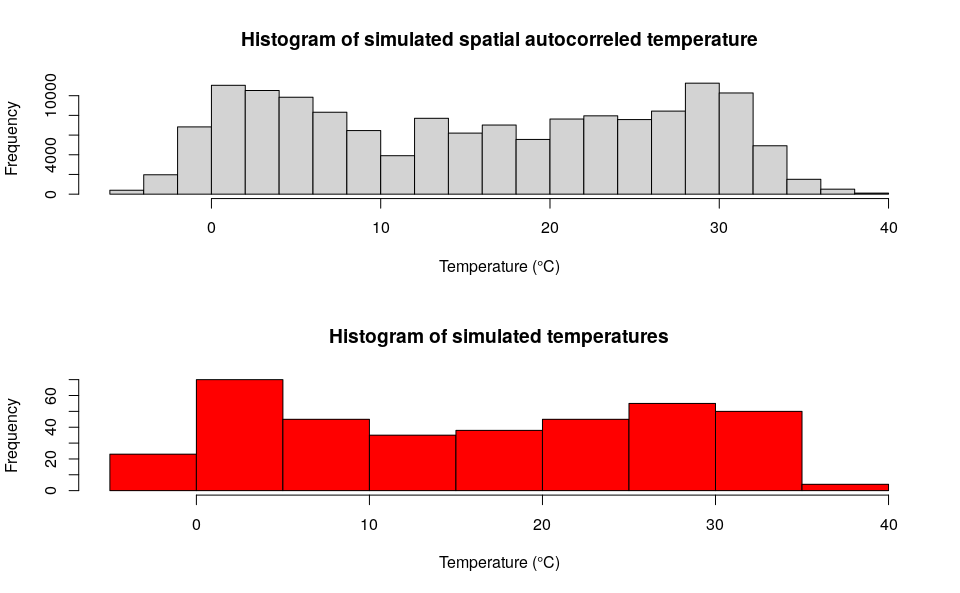par(mfrow=c(1,1))

# Format temperature data
names(mat) <- paste0("d_", 1:ndays)
df_temp <- cbind(df, mat)

## Simulate an arbitrary road segment for medium-range dispersal

In the model we have considered the possiblity of medium-range passive dispersal. Thus, we will simulate an arbitrary road segment along which adult mosquitoes can disperse passively (i.e., through car traffic).

set.seed(123)
pts <- spsample(bbox, 5, type="random")

# Check simulated segment
raster::plot(r)
raster::plot(roads, add=T)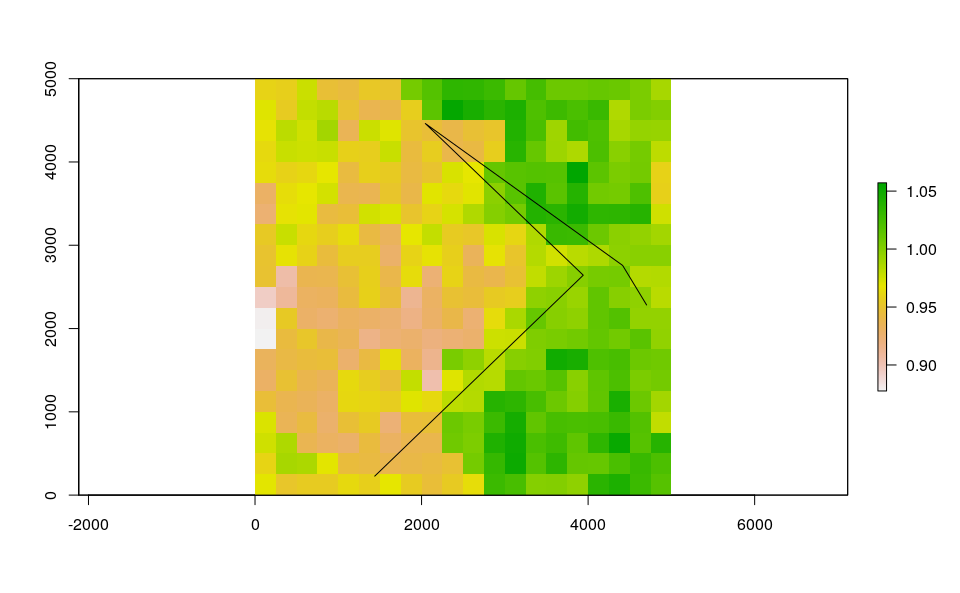After defining the road segment we add a “buffer” of 250 m around the road segment. Adult mosquitoes that reach or develop into cells comprised in the 250 m buffer around roads are thus able to undergo passive dispersal.

buff <- buffer(roads, width=250)
crs(buff) <- crs(r)
# Check grid, road segment and buffer
raster::plot(r)
raster::plot(roads, add=T, col="red")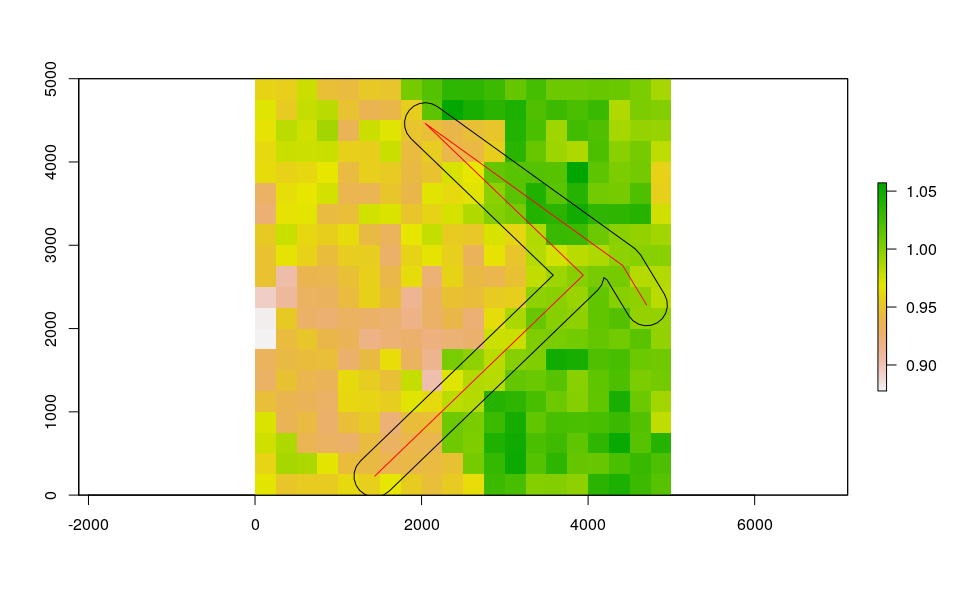Next, we derive a distance matrix between cells comprised in the spatial buffer along the road network. First, we select the cells.

df_sp <- df
coordinates(df_sp)=~x+y
df_sp <- raster::intersect(df_sp,buff)

# Check selected cells
raster::plot(r)
raster::plot(df_sp, add=T, col="red")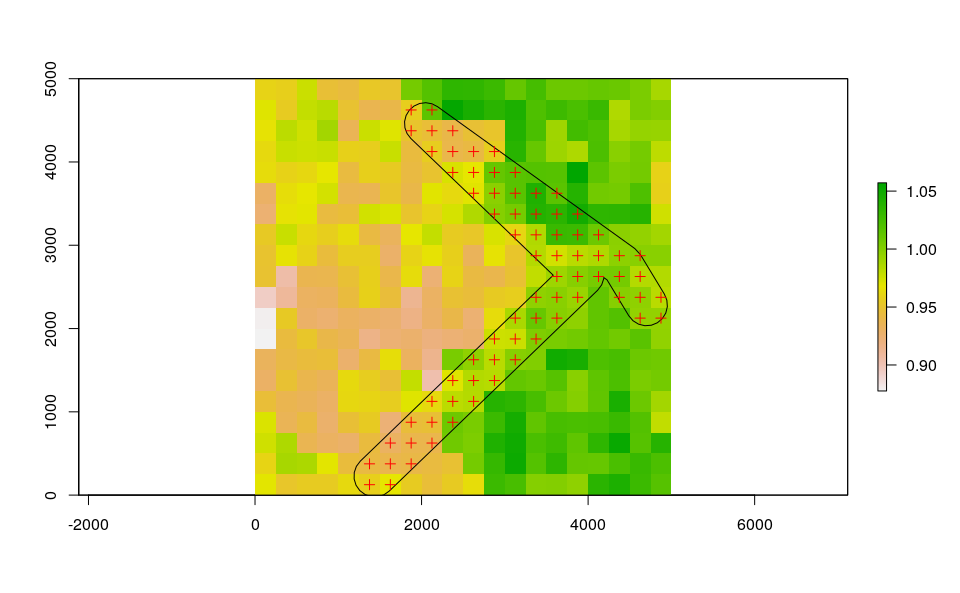Then, we compute the Euclidean distance between each selected cell.

dist_matrix <- as.matrix(dist(coordinates(df_sp)))

# Format the simulated input datasets and run the model

## Model settings

Float numbers in the temperature matrix would slow the computational speed, thus we first multiply them by 1000 and then transform them in integer numbers.

w <- sapply(df_temp[,-c(1:3)], function(x) as.integer(x*1000))

We can now define a two-column matrix of coordinates to identify each cell in the lattice grid.

cc <- df_temp[,c("x","y")]

As for model requirement, the distance matrix must have column names equal to row names.

colnames(dist_matrix) <- row.names(dist_matrix)

Moreover, distances in the distance matrix must be rounded to the thousands.

dist_matrix <- apply(dist_matrix,2,function(x) round(x/1000,1)*1000)

# An histogram showing the distribution of distances of cells along the road network
hist(dist_matrix, xlab="Distance (meters)")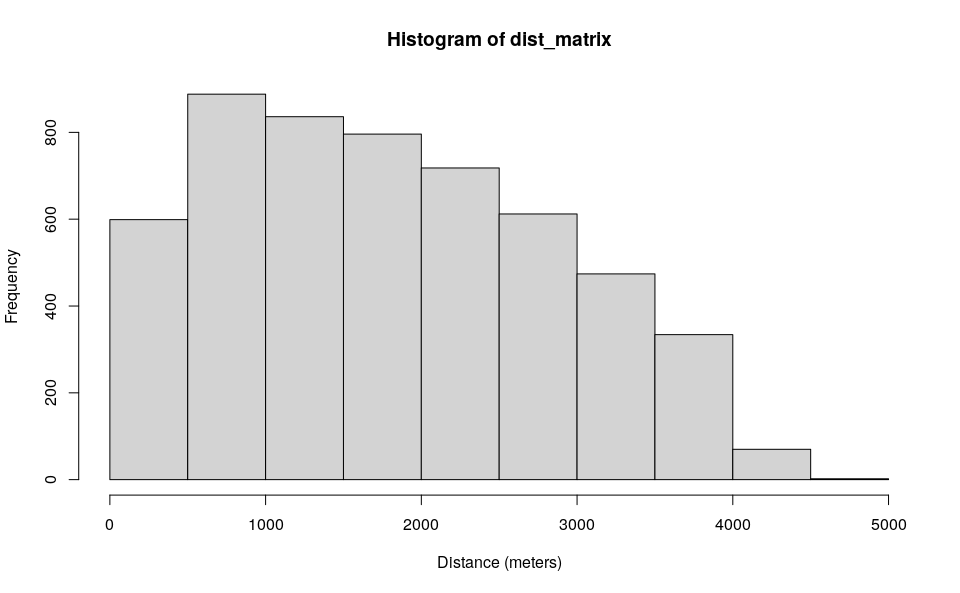Select a cell that intersect roads for introduction:

set.seed(123)
icellcoords <- df[sample(row.names(dist_matrix),1),c(2:3)]
set.seed(123)
icellid <- df[sample(row.names(dist_matrix),1),1]

raster::plot(r)
raster::plot(SpatialPoints(coords=matrix(coordinates(r)[icellid,],ncol=2)), add=T, col="black", pch=21)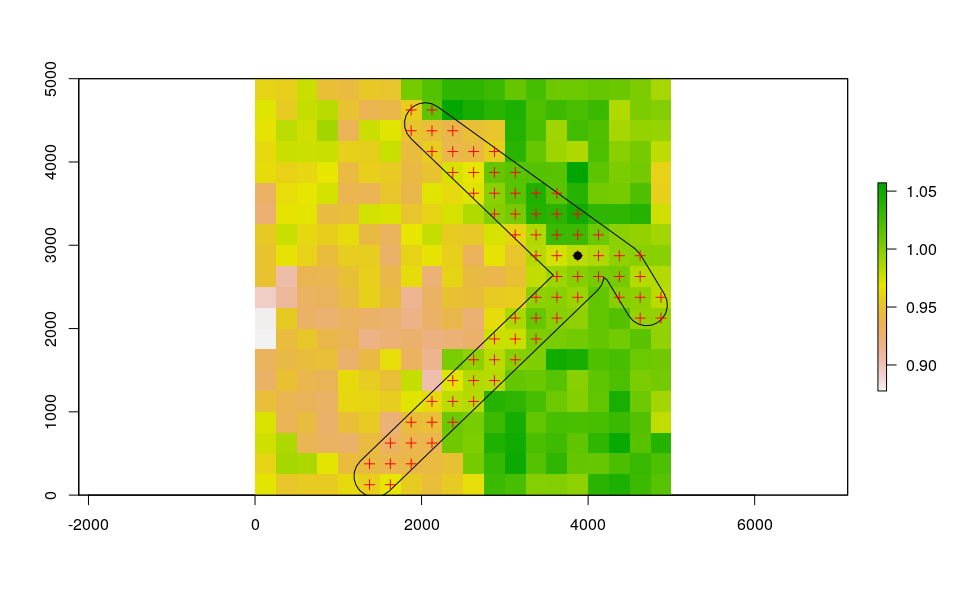We are now left with a few model variables which need to be defined.

## Define cells along roads into which introduce propagules on day 1
intro.vector <- icellid
## Define the day of introduction (August 1st is day 1)
str = 213
## Define the end-day of life cycle (October 1st is the last day)
endr = 213+(61)
## Define the number of adult females to be introduced
ia = 5000
## Define the number of model iterations
it = 1 # The higher the number of simulations the better
## Define the number of liters for the larval density-dependent mortality
habitat_liters=1
#Define latitude and longitude for the diapause process
myLat=42
myLon=7
##Define average trip distance (in km)
mypDist=2
## Define the number of parallel processes (for sequential iterations set nc=1)
cl = 1

### Run the model

Running the model with the settings specified in this example takes about 3 minutes.

simout=dynamAedes(species="albopictus",
scale="lc",
ihwv=habitat_liters,
temps.matrix=w,
cells.coords=cc,
avgpdisp=mypDist,
intro.cells=intro.vector,
startd=str,
endd=endr,
n.clusters=cl,
iter=it,
compressed.output=TRUE,
lat=myLat,
long=myLon,
cellsize=250,
dispbins=10,
seeding=TRUE,
verbose= FALSE
)

## Analyse the results

We first explore the model output structure: the simout object is a nested list.

The first level corresponds to the number of model iterations

print(it)
#  1
print(length(simout))
#  1

The second level corresponds to the simulated days. So if we inspect the first iteration, we observe that the model has computed 123 days, as we had specified above in the object endr.

length(simout[])
#  62

The third level corresponds to the amount of individuals for each stage (rows) within each grid cell of the landscape (columns). So if we inspect the first day within the first iteration, we obtain a matrix having:

dim(simout[][])
#    4 400

We can now use the auxiliary functions of the model to Analyse the results.

### Derive probability of a successfull introduction at the end of the simulated period

First, we can retrieve the probability of successful introduction, computed as the proportion of model iterations that resulted in a viable mosquito population at a given date.

psi(input_sim = simout, eval_date = 61)
#   Days_after_intro p_success      stage
# 1           Day 61         1 Population

We can also get a spatial output, using the function psi_sp, which require as additional input only the matrix of centroid coordinates of pixels.

plot(psi_sp(coords = cc, input_sim = simout, eval_date = 61, n.clusters=cl))
raster::plot(SpatialPoints(icellcoords), add=T, col="blue", pch=21)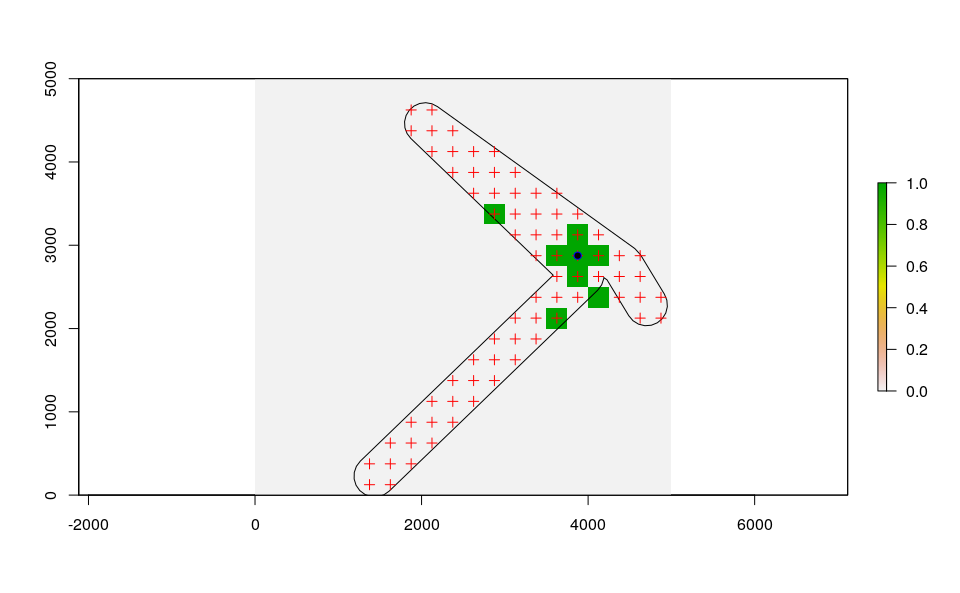At local scale, the interpretation of this output is more nuanced than for the other scales: a pixel having psi=0 can be a pixel where all the simulations resulted in an extinction or where the species has not yet arrived through dispersal.

### Derive abundance 95% CI for each life stage and in each day

We can now compute the interquantile range abundance of the simulated population over the whole landscape using the function adci.

dd <- max(sapply(simout, function(x) length(x)))#retrieve the maximum number of simulated days
egg <- as.data.frame(adci(simout, eval_date=1:dd, breaks=c(0.25,0.50,0.75), st=1))
juv <- as.data.frame(adci(simout, eval_date=1:dd, breaks=c(0.25,0.50,0.75), st=2))
eggd <- as.data.frame(adci(simout, eval_date=1:dd, breaks=c(0.25,0.50,0.75), st=4))

egg$myStage="Egg" egg$Date=seq.Date(sim_temp[]$date[str], sim_temp[]$date[endr], by="day")
juv$myStage="Juvenile" juv$Date=seq.Date(sim_temp[]$date[str], sim_temp[]$date[endr], by="day")
ad$myStage="Adult" ad$Date=seq.Date(sim_temp[]$date[str], sim_temp[]$date[endr], by="day")
eggd$myStage="Diapausing egg" eggd$Date=seq.Date(sim_temp[]$date[str], sim_temp[]$date[endr], by="day")

as_tibble()

outdf %>%
mutate(myStage=factor(myStage, levels= c("Egg", "Diapausing egg", "Juvenile", "Adult"))) %>%
ggplot( aes(y=50%,x=Date, group=factor(myStage),col=factor(myStage))) +
ggtitle("Ae. albopictus Interquantile range abundance")+
geom_line(size=1.2)+
geom_ribbon(aes(ymin=25%,ymax=75%,fill=factor(myStage)),
col="white",
alpha=0.2,
outline.type="full")+
labs(x="Date", y="Interquantile range abundance (Log10)", col="Stage", fill="Stage")+
facet_wrap(~myStage, scales = "free")+
theme_light()+
theme(legend.pos="bottom",  text = element_text(size=14) , strip.text = element_text(face = "italic"))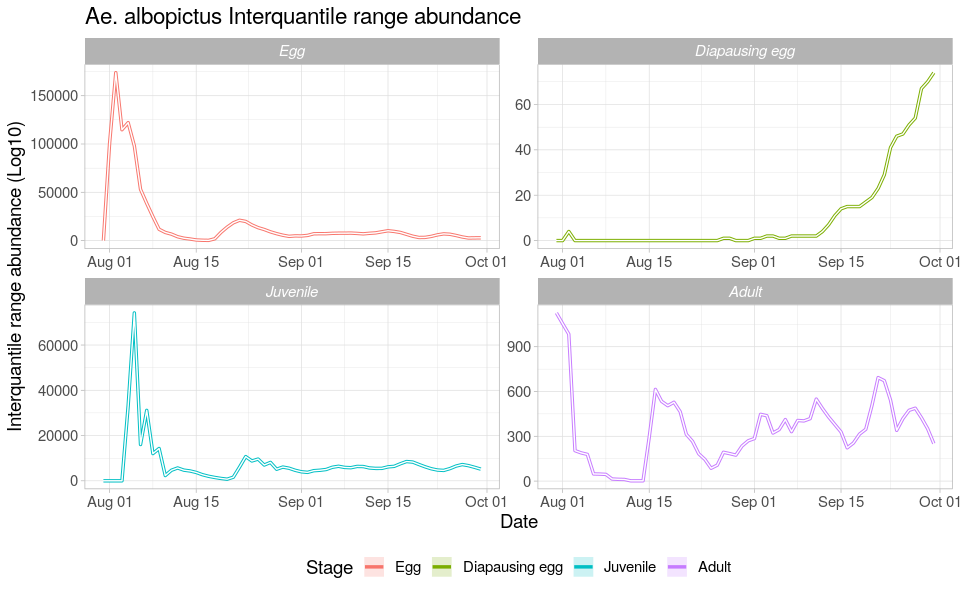We can also have a spatial output of quantiles of the abundance distribution of a given life stage and for a given simulated day by using the function adci_sp and specifiying pixel coordinates. For example for eggs:

r=adci_sp(simout, coords=cc, eval_date=61, breaks=c(0.5,0.975), stage=3)
plot(r)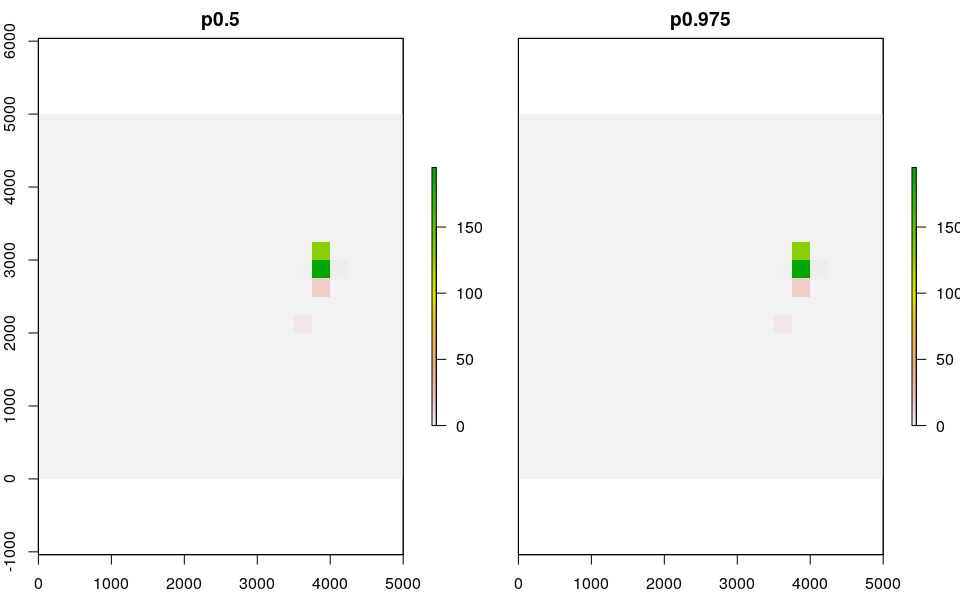Note that if only a small number of mosquitoes are present in a pixel over many iterations, quantiles may be 0 (especially for low quantiles) and you may see a series of empty rasters!

Compute a summary of the number of invaded cells over model iterations

x=icci(simout, eval_date=1:61, breaks=c(0.25,0.50,0.75))
head(x)
#    25% 50% 75% day
# V1   1   1   1   1
# V2   1   1   1   2
# V3   3   3   3   3
# V4   1   1   1   4
# V5   1   1   1   5
# V6   2   2   2   6
tail(x)
#     25% 50% 75% day
# V56   8   8   8  56
# V57   8   8   8  57
# V58   7   7   7  58
# V59   7   7   7  59
# V60   8   8   8  60
# V61   8   8   8  61

### Estimates of mosquito dispersal spread (in km2 )

Derive estimates of mosquito dispersal (in km^2) of the simulated mosquito populations (only when scale = “lc”) for any simulated day (in this case for 50 days from start and end of the simulate period).

x=dici(simout, coords=cc, eval_date=seq(1,61,length.out=61), breaks=c(0.25,0.50,0.75), space=FALSE)
plot(75%~day,x,type="l",ylab="Population dispersal (in meters) from cell of introduction",xlab="days from introduction")
lines(50%~day,x,type="l", col="red")
lines(25%~day,x,type="l")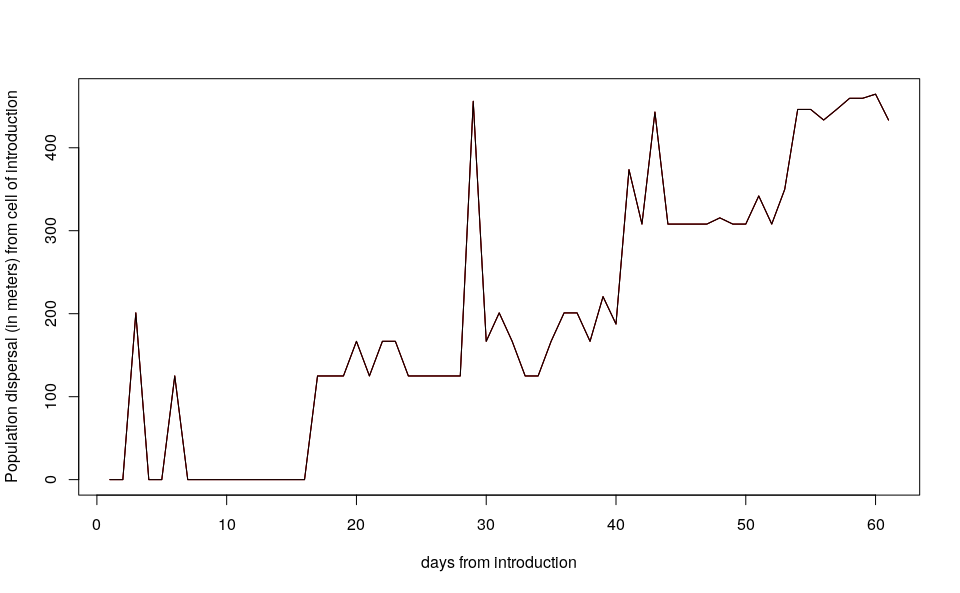# Regional scale model

The model at regional scale is the same as running the model at “punctual” scale for each cell of the grid but without accounting for active or passive dispersal. Each cell is therefore a close unit or mosquito population. With this setting, the model requires two input datasets: a numerical temperature matrix (in degree Celsius) defined in space and time (space in the rows, time in the columns), and a two-column numerical matrix reporting the centroid coordinates (in meters) of each cell. For the purpose of this tutorial, we will use the following simulated datasets:

1. A 5 km lattice grid with 250 m cell size;
2. A 1-year long spatially and temporally correlated temperature time series;

## Model settings

We take advantage of the spatial temperature dataset simulated for the local scale example.

Float numbers in the temperature matrix would slow the computational speed, thus we first multiply them by 1000 and then transform them in integer numbers.

w <- sapply(df_temp[,-c(1:3)], function(x) as.integer(x*1000))

We can now define a two-column matrix of coordinates to identify each cell in the lattice grid.

cc <- df_temp[,c("x","y")]

We are now left with a few model variables which need to be defined.

## Define the day of introduction (June 1st is day 1)
str = 152
## Define the end-day of life cycle (October 1st is the last day)
endr = 152+(61*2)
## Define the number of eggs to be introduced
ie = 100
## Define the number of model iterations
it = 1 # The higher the number of simulations the better
## Define the number of liters for the larval density-dependent mortality
habitat_liters=1
#Define latitude and longitude for the diapause process
myLat=42
myLon=7
## Define the number of parallel processes (for sequential iterations set nc=1)
cl = 1

### Run the model

Running the model with the settings specified in this example takes about 3 minutes.

simout=dynamAedes(species="albopictus",
scale="rg",
ihwv=habitat_liters,
temps.matrix=w,
cells.coords=cc,
startd=str,
endd=endr,
n.clusters=cl,
iter=it,
intro.eggs=ie,
compressed.output=TRUE,
lat=myLat,
long=myLon,
verbose = FALSE)

## Analyse the results

We first explore model output structure: the simout object is a nested list.

The first level corresponds to the number of model iterations

print(it)
#  1
print(length(simout))
#  1

The second level corresponds to the simulated days. If we inspect the first iteration, we observe that the model run for 123 days, as we had specified above in the object endr.

length(simout[])
#  123

The third level of the output list object corresponds to the amount of individuals for each stage (rows) within each grid cell of the landscape (columns). If we inspect the first day within the first iteration, we obtain a matrix having

dim(simout[][])
#    4 400

We can now use the auxiliary functions of the package to Analyse the results.

### Derive probability of a successfull introduction at the end of the simulated period

First, we can retrieve the “probability of a successful introduction”, computed as the proportion of model iterations that resulted in a viable mosquito population (in any cells of the grid) at a given date.

psi(input_sim = simout, eval_date = 123)
#   Days_after_intro p_success      stage
# 1          Day 123         1 Population

We can also get a “spatial output”, using the function psi_sp, which requires as additional input only the matrix of the pixels coordinates

plot(psi_sp(coords = cc, input_sim = simout, eval_date = 123,n.clusters=cl))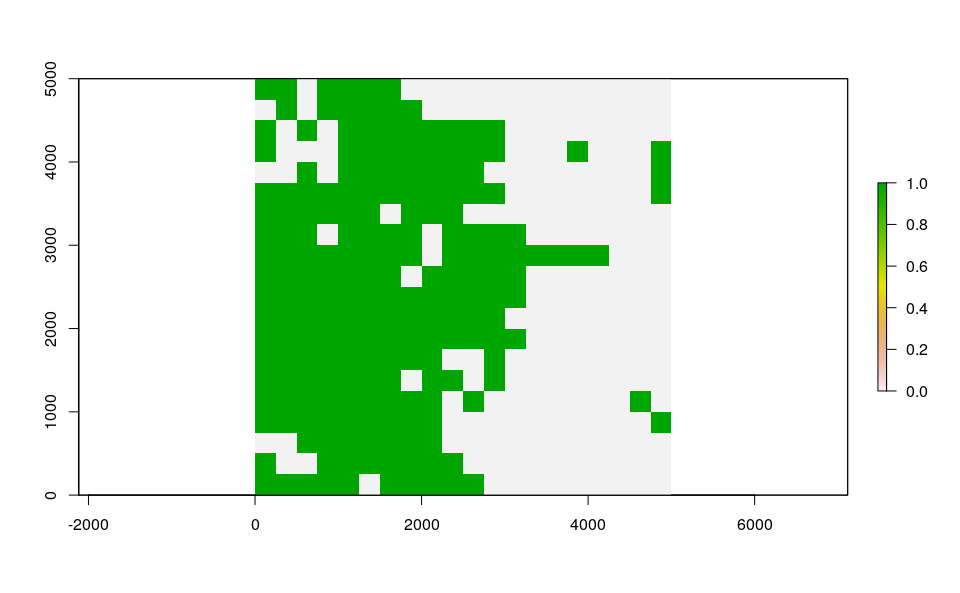### Derive abundance 95% CI for each life stage and in each day We can now compute the interquantile range abundance of the simulated population using the function adci over the whole landscape.

dd <- max(sapply(simout, function(x) length(x)))#retrieve the maximum number of simulated days
egg <- as.data.frame(adci(simout, eval_date=1:dd, breaks=c(0.25,0.50,0.75), st=1))
juv <- as.data.frame(adci(simout, eval_date=1:dd, breaks=c(0.25,0.50,0.75), st=2))
eggd <- as.data.frame(adci(simout, eval_date=1:dd, breaks=c(0.25,0.50,0.75), st=4))

egg$myStage="Egg" egg$Date=seq.Date(sim_temp[]$date[str], sim_temp[]$date[endr], by="day")
juv$myStage="Juvenile" juv$Date=seq.Date(sim_temp[]$date[str], sim_temp[]$date[endr], by="day")
ad$myStage="Adult" ad$Date=seq.Date(sim_temp[]$date[str], sim_temp[]$date[endr], by="day")
eggd$myStage="Diapausing egg" eggd$Date=seq.Date(sim_temp[]$date[str], sim_temp[]$date[endr], by="day")

as_tibble()

outdf %>%
mutate(myStage=factor(myStage, levels= c("Egg", "Diapausing egg", "Juvenile", "Adult"))) %>%
ggplot( aes(y=(50%),x=Date, group=factor(myStage),col=factor(myStage))) +
ggtitle("Ae. albopictus Interquantile range abundance")+
geom_line(size=1.2)+
geom_ribbon(aes(ymin=25%,ymax=(75%),fill=factor(myStage)),
col="white",
alpha=0.2,
outline.type="full")+
labs(x="Date", y="Interquantile range abundance", col="Stage", fill="Stage")+
facet_wrap(~myStage, scales = "free")+
theme_light()+
theme(legend.pos="bottom",  text = element_text(size=14) , strip.text = element_text(face = "italic"))